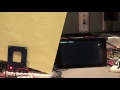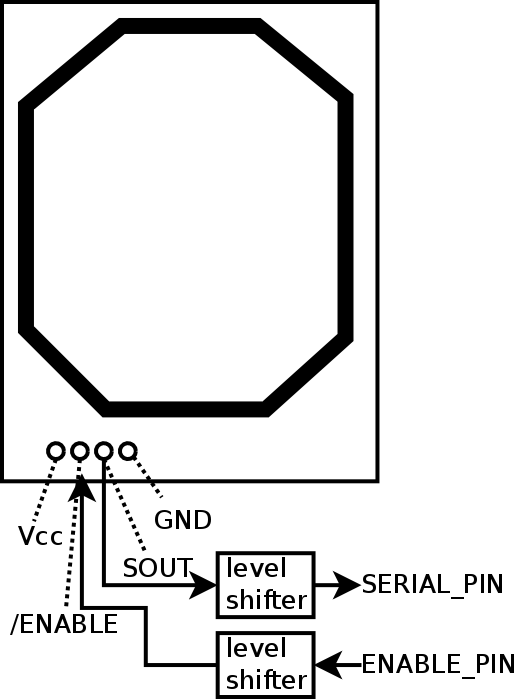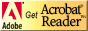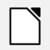# Raspberry Pi Interface with Parallax Serial RFID Reader

Demonstration## Physical connections### Notes

1. VCC for the reader is 5V, so level shifting is needed for the Raspberry Pi.
2. Because the reader uses a baud rate of only 2400bps, a bit-banged solution is possible and leaves the Raspberry Pi serial port untouched.
3. Any GPIO pins can be used. I used GPIO5 for /ENABLE and GPIO27 for SOUT.
4. The reader can send repetitions of the code for one swipe of a tag; it seems to be pretty random.

## Python code

```#!/usr/bin/python
# Bitbanging code to use Parallax serial RFID reader
# Terry Sturtevant, May 9, 2017

import RPi.GPIO as GPIO
from datetime import datetime
import time

GPIO.setmode(GPIO.BCM)
ENABLE_PIN=5

#This could presumeably be modified for other serial devices
PORT_RATE=2400
BYTESIZE=8
PARITY="N"
STOP_BITS=1
END_LINE='\n'

# any GPIO pin can be used
SERIAL_PIN=27
START_LEVEL=False
STOP_LEVEL=True

HALF_BIT_TIME=1/float((2*PORT_RATE))
THREE_HALF_BIT_TIME=3*HALF_BIT_TIME
ONE_BIT_TIME=2*HALF_BIT_TIME
HALF_BIT_TIME_US=int(HALF_BIT_TIME*1000000)
THREE_HALF_BIT_TIME_US=int(1000000*THREE_HALF_BIT_TIME)
ONE_BIT_TIME_US=int(1000000*ONE_BIT_TIME)

#in seconds

CHAR_TIME_US=(BYTESIZE*2+STOP_BITS)*HALF_BIT_TIME_US
message=[]
chartimes=[]

#In order to be fast enough in Python, this routine just generates an array
# of transition times on the serial pin (in microseconds)
# At the beginning of each new character, the time is reset to zero
# (Number of transitions for a character must be odd)
##
def timeChar():

timestart=0
times=[]

# wait for start bit
input_value=GPIO.input(SERIAL_PIN)
while input_value != START_LEVEL:
input_value=GPIO.input(SERIAL_PIN)
# get data  transition time of start bit

dt=datetime.now()
nowus=dt.microsecond
times.append(nowus)
timestart=nowus
#calculate time for the entire character
timeend=timestart+CHAR_TIME_US

# get data  transition time of other bits
# stop when the time for the entire character has passed
while nowus<timeend:

oldLevel=input_value
input_value=GPIO.input(SERIAL_PIN)

while input_value == oldLevel:
input_value=GPIO.input(SERIAL_PIN)

dt=datetime.now()
nowus=dt.microsecond
times.append(nowus)

times.append(times)

# wait for stop bit

input_value=GPIO.input(SERIAL_PIN)
while input_value != STOP_LEVEL:
input_value=GPIO.input(SERIAL_PIN)

#now make times relative to the start of the character
#so the start bit is at 0, by definition

temptime=times

for i in range(len(times)):
times[i]=times[i]-temptime

return times

# given an array of transition times, and knowing the time for a bit,
# and that the character starts with a START bit, the complete
# bit string can be generated
# The data comes in LSB first, so it gets reversed at the end
##
def convertChar(thesetimes):
bytestr=''
curtime=0
curLevel='1'
altLevel='0'
curBit=0

if thesetimes<CHAR_TIME_US:

for i in range(len(times)):
while thesetimes[i]>curtime+THREE_HALF_BIT_TIME_US:
bytestr+=curLevel
curtime+=ONE_BIT_TIME_US
curBit+=1
tempLevel=curLevel
curLevel=altLevel
altLevel=tempLevel

return bytestr[::-1]

####################MAIN##################

#beginning of main code

#The reader has an ENABLE pin, which must be LOW to read
GPIO.setup(ENABLE_PIN,GPIO.OUT)
GPIO.setup(SERIAL_PIN,GPIO.IN)

#This just makes a flash of the LED
GPIO.output(ENABLE_PIN,GPIO.HIGH)
time.sleep(0.5)
GPIO.output(ENABLE_PIN,GPIO.LOW)

try:

charSpace=0
msgIndex=1
startTime=datetime.now()
msgTime=startTime-startTime

msgStarted=False
msgDone=False

#A message will be assumed to be complete if there
# hasn't been a transition in ALLOWABLE_DEAD_TIME (seconds)
#Processing of transisiton times isn't done until AFTER
# the message is complete to allow a higher baud rate
while not msgDone:
#wait for activity
input_value=GPIO.input(SERIAL_PIN)
if input_value == STOP_LEVEL:
thisTime=datetime.now()
if not msgStarted:
startTime=thisTime
msgTime=thisTime-startTime
msgDone=True
else:
# get a character
chartimes=timeChar()
startTime=datetime.now()
msgStarted=True

message.append(chartimes)
msgIndex+=1

#message is done, so disable reader
GPIO.output(ENABLE_PIN,GPIO.HIGH)

#now process transition times
charstr=''
for i in range(msgIndex-1):

times=message[i]
value=convertChar(times)
ascval= int(value,2)
charstr+= chr(ascval)
times=[]

print charstr

except KeyboardInterrupt:
pass

GPIO.cleanup()
```

### Notes

1. Quantities ending in _US are in microseconds.
2. In this application, where there are big gaps in activity on the serial line when tags are not being read, it works fine. If there were more continuous activity timing could be a problem. This could probably be adapted for slightly higher baud rates, (e.g. 4800 and maybe 9600), but probably not much higher.

## ResourcesIf you need to update a browser, you might try Firefox which is
• free
• open source
• available for several platformsSince this page uses cascading style sheets for its layout, it will look best with a browser which supports the specifications as fully as possible.

If you are looking for an office package, with a word processor, spreadsheet, etc., you might try LibreOffice which is
• free
• open source
• available for several platformsGo to the main page for the Department of Physics and Computer Science.

Wilfrid Laurier University